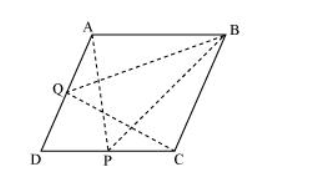# P and Q are any two points lying on the sides DC and AD respectively of a parallelogram ABCD.

Question. P and Q are any two points lying on the sides DC and AD respectively of a parallelogram ABCD. Show that ar (APB) = ar (BQC).

Solution:It can be observed that ΔBQC and parallelogram ABCD lie on the same base BC and these are between the same parallel lines AD and BC.

$\therefore$ Area $(\triangle B Q C)=\frac{1}{2}$ Area $(A B C D) \ldots$(1)

Similarly, ΔAPB and parallelogram ABCD lie on the same base AB and between the same parallel lines AB and DC.

$\therefore$ Area $(\triangle A P B)=\frac{1}{2}$ Area $(A B C D)$..(2)

From equation (1) and (2), we obtain

Area $(\Delta B Q C)=$ Area $(\triangle A P B)$